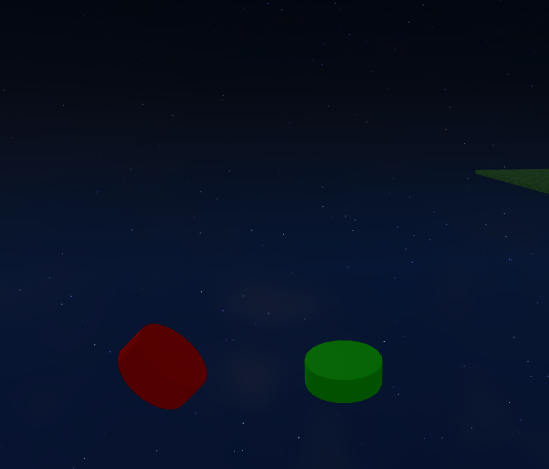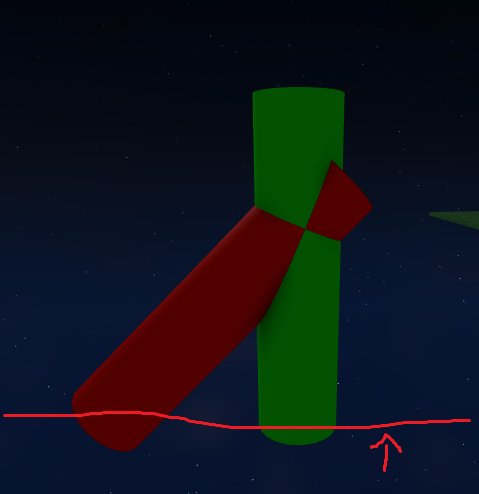# Any way to make sure that two cylinders (with orientation) aren't intersecting?

Let’s say I have two cylinders.I want to make sure that when these two cylinders grow in length, they won’t ever overlap.This is all I have so far, but it doesn’t seem to work. Mind you I’m not very good at CFrame.

``````RedCylinder.CFrame = GreenCylinder.CFrame * CFrame.new(0, (GreenCylinder.Size.Y / 2 - (GreenCylinder.Size.Y * self.YSpawn)), 0)
-- Gets where the red cylinder should spawn, not accurate to the image above sorry.

RedCylinder.CFrame = CFrame.lookAt(RedCylinder.Position, GreenCylinder.Position - Vector3.new(math.random(-360, 360),math.random(-360, 360),math.random(-360, 360)))
-- Makes the RedCylinder look away from the GreenCylinder and adds random orientation, doesn't work 100% of the time however.
``````

Just trying to make the RedCylinder be able to greow in the same general direction (in this case upwards) but also not ever intersect with the GreenCylinder. Or any other RedCylinders in the future that might be added?

Any help is appreciated. My current method is just to have the RedCylinder look away from the GreenCylinder and add random orientation, however it doesn’t always work.imagine the cylinders as two rays with origin `p.Position` and direction `p.CFrame.XVector`. you can make a couple of observations:

• the rays have to be at least 2R studs away from each other at all times where R is the radius of the cylinders
• the line segment of minimum possible distance between these rays is perpendicular to both of them

perpendicular to both rays, well that’s just cross product.

``````local v = R.CFrame.XVector:Cross(G.CFrame.XVector)
``````

we can use v as the normal for two planes using the positions of both cylinders and find the distance between both of them since they are parallel.

``````local d1, d2 = -(v.X*R.Position.X+v.Y*R.Position.Y+v.Z*R.Position.Z), -(v.X*G.Position.X+v.Y*G.Position.Y+v.Z*G.Position.Z)

local dist = math.abs(d1-d2)/v.Magnitude
local willIntersect = dist < (R.Size.Z/2 + G.Size.Z/2)
``````

if the cylinders are thin enough I’d just recommend looping random directions until `willIntersect == false`.

2 Likes

Works great!Thanks for explaining in detail!

Not sure how to ask this question, but how can I make the orientation/math you sent be relative to its current orientation?

Both green and red cylinders can generate on any surface normals. So the math you sent does work for cylinders that generate on the ceiling, however ones that generate on the walls or floor become inverted.Imagining if the red line was a floor that the cylinders shouldn’t grow into, how can I always have the cylinders generate facing up from it?

I use :Raycast() to actually get the cylinder spawning positions, do I need to use RaycastResult.Normal for this?

1 Like

are they still colliding with each other or just not growing in the right direction? I think it should work for any orientation. probably. that’s me talking, though.

to make sure they grow towards the normal I would try

``````local rng = Random.new()
local root = CFrame.lookAt(rc.position, rc.position + rc.Normal)
``````

perhaps unorthodox, but I often use CFrame.lookAt + ToWorldSpace because I’m bad at math. you might need to mess with the CFrame.Angles order a bit, I kind of just guessed.

1 Like

I’ll try this out in a sec, thanks for this!

Also to answer, they are not colliding, just not growing in the right direction.

You’re seriously a genius, I may not understand any of the code but it does work. Thank you so much.

1 Like

One slight thing, the red cylinders all end up pointing the same way, even when tweaking the math.rad generator.

``````--SplitBase is the red cylinder while  ParentBase is the green.
SplitBase.CFrame = ParentBase.CFrame * CFrame.new(0, (ParentBase.Size.Y / 2 - (ParentBase.Size.Y * self.SplitYSpawn)), 0)

local Attempts = 0

repeat

local PlaneNormals = SplitBase.CFrame.XVector:Cross(ParentBase.CFrame.XVector)

Attempts += 1
until (math.abs((PlaneNormals.X * SplitBase.Position.X + PlaneNormals.Y * SplitBase.Position.Y + PlaneNormals.Z * SplitBase.Position.Z) + (PlaneNormals.X * ParentBase.Position.X + PlaneNormals.Y * ParentBase.Position.Y + PlaneNormals.Z * ParentBase.Position.Z)) / PlaneNormals.Magnitude < (SplitBase.Size.Z / 2 + ParentBase.Size.Z / 2)) == false or Attempts >= 3

if Attempts >= 3 then
return
end
``````

Should I be randomizing the RaycastResult.Normal? Not sure what to do

that is very strange, are you sure you’re not accidentally using the same rng state each time (i.e. Random:Clone(), Random.new(tick()) in the same frame)? that’s all I could think of.